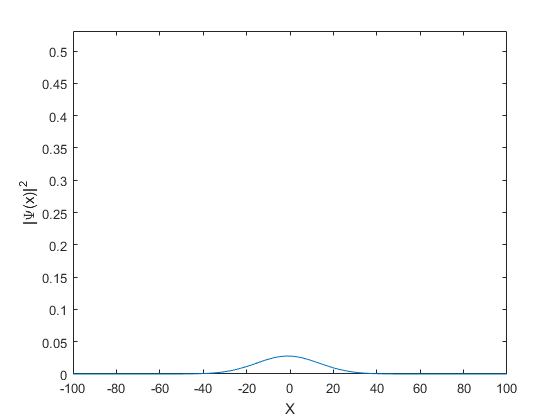# Particle Wave Packets

A particle in free space who's momentum is known exactly can be described by the plane wave probability amplitude function:

## Contents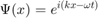where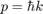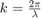and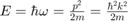We will consider a particle that is localized in space with a Gaussian probabilty amplitude distribution at t=0 is:Our appoach is to project this wave packet onto the plane wave solutions, then let each plane wave evolve as \$e^{i( k x - \omega t)}%

## Lets make a wave packet

Lets take hbar=1, m=1

N=201;
X=linspace(-100,100,N);
DX=X(2)-X(1);
Sigma=2;
A=normpdf(X,0,Sigma);
A=A/sqrt(A*A'*DX); %Normalize
figure
plot(X,A)
xlabel('X');ylabel('\Psi(x)')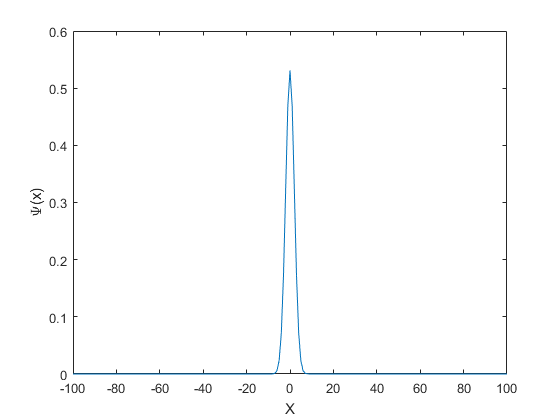## Write wave packet as a sum of plane waves

% Expanding into plane waves is just the Fourier transform:
Y=fftshift(fft(A));
K=(0:N-1);
K(K>N/2)=K(K>N/2)-N;
K=fftshift(K);

% Plot the wave in k-space (this is spatial frequency)
figure
plot(K,real(Y))
hold
plot(K,imag(Y))
plot(K,abs(Y),'k','linewidth',2)
legend('real','imag','abs')
xlabel('K');ylabel('Y(K)')
% Note the magnitude of the Y(k) is also a Gaussian.
% The FT of a Gaussian is a Gaussian.
% web('http://mathworld.wolfram.com/FourierTransformGaussian.html')

% Plot as wave number with correct units
figure
DK=1/(N*DX);
KWV=2*pi*K*DK;     %Wave number
plot(KWV,real(Y))
hold
plot(KWV,imag(Y))
plot(KWV,abs(Y),'k','linewidth',2)
legend('real','imag','abs')
xlabel('K');ylabel('Y(K)')

Current plot held
Current plot held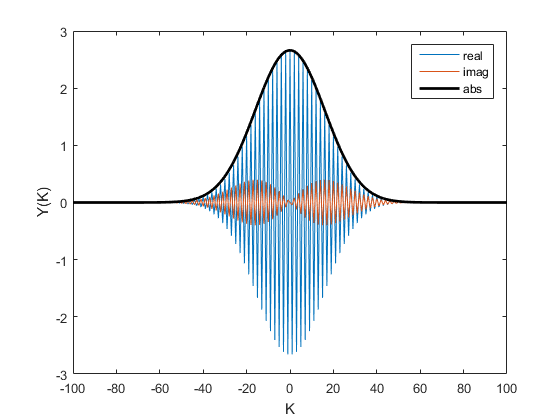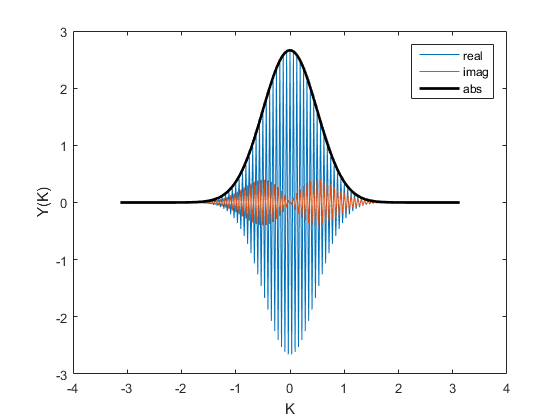Now let's reconstruct the wave from Fourier coefficients at t=0

close all
Wave=0
figure
for kk=1:N
KS=K(kk);           %Spatial frequency
KWV=2*pi*KS;        %Wave number
PlaneWave=1/N*exp(i*KWV*(0:N-1)/N);
Wave=Wave+Y(kk)*PlaneWave;
plot(X,real(Wave))
xlabel('X');ylabel('\Psi(x)')
pause(.01)
end

Wave =

0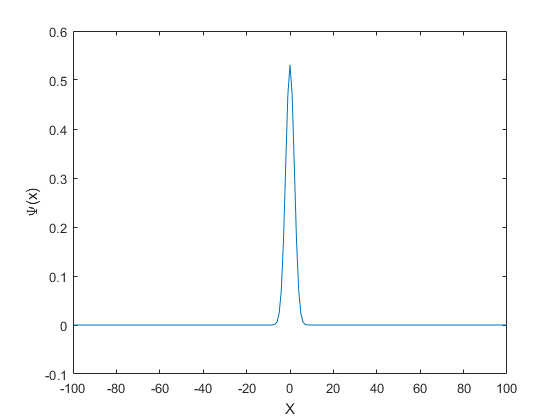Now let's look at time evolution

Tvec=linspace(0,.001,100);
figure
for tt=1:length(Tvec)
Wave=0;
t=Tvec(tt);
for kk=1:N
KS=K(kk);           %Spatial frequency
KWV=2*pi*KS;        %Wave number
W=1/2*KWV^2;        %Omega
PlaneWave=Y(kk)/N*exp(i* (KWV/N*(1:N)-W*t) );
Wave=Wave+PlaneWave;
end
plot(X,abs(Wave).^2)
axis([-100 100 0 max(A)])
xlabel('X');ylabel('|\Psi(x)|^2')
pause(.01)
end